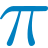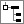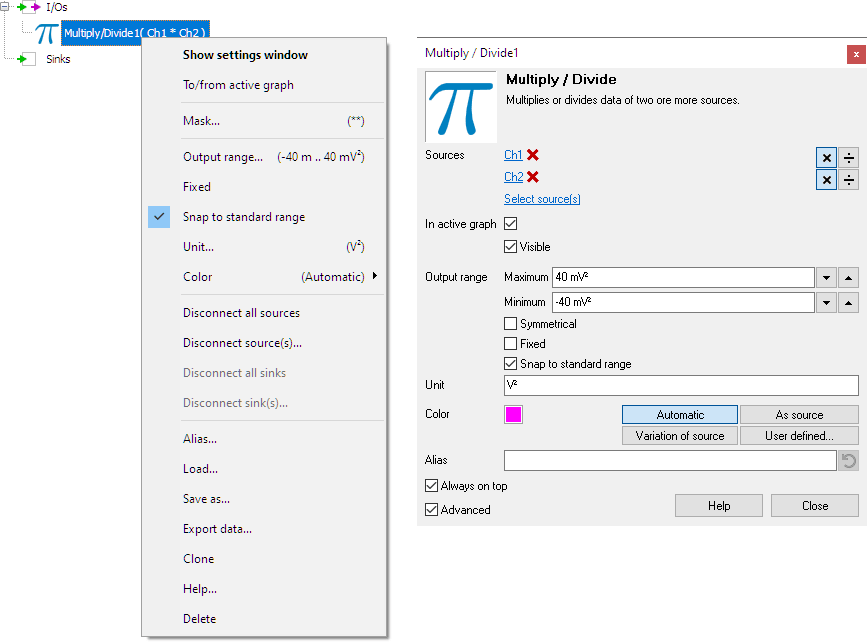# Multiply / DivideThe Multiply / Divide I/O multiplies or divides data of two ore more sources. Up to 32 sources can be connected to a Multiply / Divide I/O.

A typical application of the Multiply / Divide object is power measurement. When you measure the voltage over a load and the current through it, you can calculate the power by multiplying both measurements.

## Properties

To control the behavior of the Multiply / Divide I/O, several properties are available. These can be accessed through a popup menu which is shown when the I/O is right clicked in the Object Tree. The properties can also be accessed through its settings window which is shown when the I/O is double clicked in the Object Tree. To open the Object tree, click theShow object tree button.By default, the settings window only shows the most used settings. When Advanced is ticked, the extended window with all settings is shown. See also the program settings.

The Mask determines which sources are in the nominator (default) and which sources are in the denominator. This mask contains a '*' or '/' character for every connected source. By default, the mask consists of '*'-es only and all sources are multiplied. To divide by a source, set its corresponding mask character to '/'.

Some examples of */ masks are shown in the table below:

1 / 1 / src1
2 ** src1 * src2
2 */ src1 / src2
2 /* src2 / src1
2 // 1 / (src1 * src2)
3 *// src1 / (src2 * src3)
6 */*//* (src1 * src3 * src6) / (src2 * src4 * src5)

### Related information

#### Software generator

The Software generator source (SofGen) generates fully configurable standard signals like sine, block and triangle.

#### Gain / Offset

The Gain / Offset I/O multiplies a signal with a constant gain factor and adds a constant offset.

The Add / Subtract I/O adds or subtracts data of two or more sources.

#### Sqrt

The SQRT I/O calculates the square root of each sample of the source's data.

#### ABS

The ABS I/O takes the absolute value of each sample of the source's data.

#### Differentiate

The Differentiate I/O differentiates the source's data.

#### Integrate

The Integrate I/O integrates its source's data.

#### Logarithm

The Logarithm I/O calculates the logarithm of the source's data.